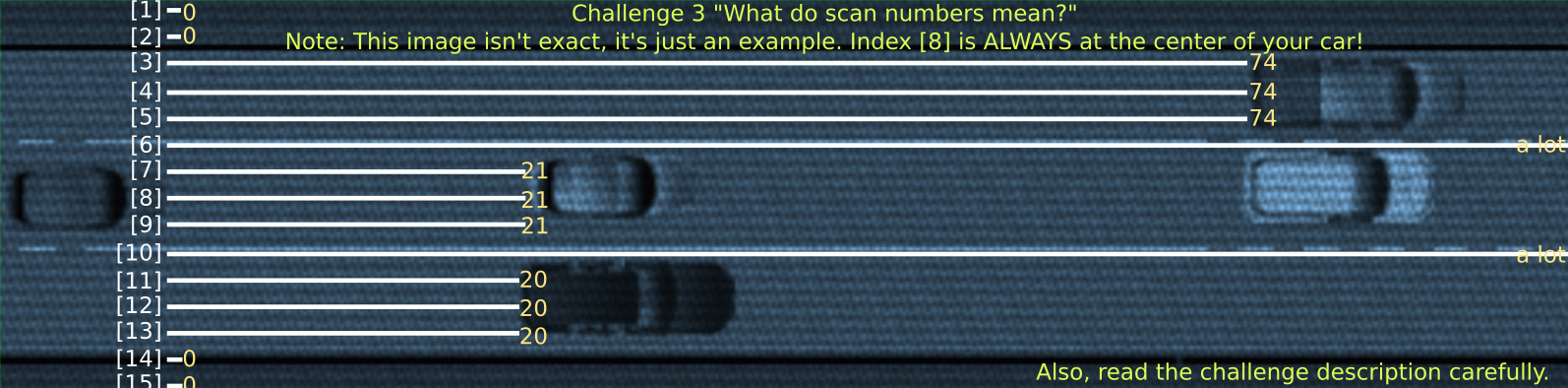WebScripting

1 year ago - 295 views

# 3 - High Speed Chase

This challenge again gives us a link to a website. On this website is an area to write javascript code on the left, with some instructions on the right.
On the top we also see 3 lanes with cars, this is the challenge we need to get through. This is more of a coding challenge than a security challenge. But it is still fun to think of creative algorithms to solve it.

## The Challenge

The goal of this challenge is to guide the car through the traffic without hitting other cars, or the curb of the road.
We get an array (`scanArray`) with 17 values which represent parallel lines 'scanning' the view in front of the car. The lines move with the car so that index 8 is always in the center of our car.Then after getting this information in our function, we need to return a value that tells the car to go left, right, or to do nothing.
The value `-1` represents left, or up if you look at it from the side like on the page. Then `1` represents right, or down. Lastly `0` represents doing nothing, this is also what happens if you don't return anything in your function.

## The Idea

After thinking and trying a lot of different approaches, I thought of a pretty simple and effective idea. I thought to myself, what would a human do?
You look at where there is enough room for your car to go through, and how far down it goes. Then the best path to go is a combination of both.

By going through the `scanArray` with slices of 3 (the width of our car) we can look at every lane the car could go through, and how far it goes. Multiplying all these distances together gets a good value to compare against other possible lanes.
For example: if there is a lane that has 2 lines going 100m, but 1 line that is only going 10m until a car, the total score for this lane is `100*100*10 = 100.000`. Now imagine another lane that has 3 lines going 50m. That would result in a value of `50*50*50 = 125000`. A higher score than the other lane, which makes sense because we don't want to try and go to a lane where there is only 10m until the next car. That is way too risky. This technique prefers consistent high numbers.
One more thing about this idea is the fact that the curb is counted as a distance of 0. Multiplying anything by 0 results in 0. So our total, if we would hit the curb, would always be 0, making sure we never it the curb.

## Solution

Implementing this idea into JavaScript code for the challenge is pretty simple.
First, we need to be able to slice the array into lengths of 3.
`scanArray.slice(i, i+3)` and incrementing `i` by 1 every time gives us every possible lane. Then we can multiply all these values together to get our total score.
We can use `.reduce((total, current) => total * current);` to mutiply all numbers with each other.
Every iteration we need to compare the total score to our best yet, to see if this beats it. Later we need the index as well to move the car in the right direction so we need to save that as well.
Finally, we need to look at what direction the car needs to drive in. Looking at the index we saved earlier we can see if it is before or after the center of our car (8). If it is less than the center, that means we need to go left, because that is the direction of our best lane. And if it is more than our center we need to go right.

Combining all this finally gets us the following code:

JavaScript

``````const width = 3;

var bestTotal = 0;
var bestIndex = -1;

for (var i = 0; i < scanArray.length-width; i++) {
// Multiply distances together
var total = scanArray.slice(i, i+width).reduce((total, current) => total * current);

// Save if new best
if (total > bestTotal) {
bestTotal = total;
bestIndex = i+Math.floor(width/2);  // Set index to center of lane
}
}

if (bestIndex < 8) {
return -1;  // Left
} else if (bestIndex > 8) {
return 1;  // Right
} else {
return 0;  // Nothing
}``````

Running this code in the simulator dodges all the cars and eventually gives us the flag!
`CTF{cbe138a2cd7bd97ab726ebd67e3b7126707f3e7f}`